# Lecture 11: Slice Sampling¶

AM207: Pavlos Protopapas, Harvard University

March 4, 2014

In :
%matplotlib inline
import numpy as np
import scipy as sp
import matplotlib.pyplot as plt
import pandas as pd
pd.set_option('display.width', 500)
pd.set_option('display.max_columns', 100)
import seaborn as sns

from scipy.stats import norm

import sys
from IPython import display

$$\newcommand{\var}{{\rm var}} \newcommand{\cov}{{\rm cov}} \newcommand{\corr}{{\rm corr}} \newcommand{\xss}{x^{(i+1)}} \newcommand{\xs}{x^{\star}} \newcommand{\xt}{x^{(i)}} \newcommand{\yss}{y^{(i+1)}} \newcommand{\ys}{y^{\star}} \newcommand{\yt}{y^{(i)}} \newcommand{\xa}{x_a} \newcommand{\xb}{x_b}$$

# Introduction¶

We've already studied two MCMC variants, Gibbs Sampling and Metropolis-Hastings. These are the foundational (and in many senses, original) MCMC methods. Both methods are based on random walk Markov Chains. You may have started to notice, or at least suspect, that there are many situation in which we want greater flexibity in exploring the probability space than waht a random walk Markov Chain provides. There are a variety of MCMC methods that offer different approaches to traversing the distribution than Metropolis-Hastings (or Gibbs Sampling which is after all a type of Metropolis-Hastings). We'll explore a number of these methods over the next few weeks.

One of the methods that we'll look at is Slice Sampling. Slice sampling was invented by Radfor Neal and John Skilling. It is a very elegant and simple way to solve the problems of MH and Gibbs sampling. So easy, that you would think my grandmother invented it. Slice Sampling is geared to approach the following problem with Methropolis-Hastings. Metropolis Hastings is very sensitive to the width of the proposal step. You can tune the Metropolis-Hastings algorithm by choosing a proposal in such a way as to determine the width of the proposal distribution for each variable. Unfortunately satisfying detailed-balance provides a constraint preventing any proposal width scale parameters from being set based on past iterations of the current chain.

One can set the proposal distribution width based on prior knowledge (usually done by running trial chains), but will often be confronted with the choice between a large width that explores the space efficiently but results in many rejections or a small proposal width that has a low rejection rate but explores the parameter space inefficiently. We usually want the width (aka step) to be as large as possible but not that large. Gibbs sampling does not have those parameters and therefore we may like it, but it only works when we know the conditional distribution.

## How does slice sampling work?¶

If you recall from Data Augmentation, if we have a posterior distribution (i.e. X ~ p(x) ) from which we're trying to sample, we can augment that distribution with additional variables to create a joint distribution p(x,y) with conditional distributions Y|X and X|Y that are easy to sample from. We can use Gibbs to sample from these conditional distributions and keep the X samples while eventually discarding the Y samples that we don't need.

Slice sampling takes advantage of this same methodology to to try to efficiently sample from a distribution while dynamically adapting step-size to the local region it's sampling. Let's take a look how.

### The General Univariate Slice Sampling Algorithm¶

1. Inititalize by randomly selecting $x^{(k)}$ where $k=0$
2. Draw $y^{(k)}$ from $U(0, f(x^{(k)}))$
3. Find an interval I = (L, R) around $x^{k}$ corresponding to $S = \{x\, s.t.\, f(x) > y^k \}$
4. Draw $x^{(k+1)}$ from U(I)
5. Repeat from (2) onwards

Note, $S$ is the perfect region defined in (2) and $I$ is the interval we choose to correspond to this region.

In :
mu1=3; mu2=10; sigma1=1; sigma2=2; l1=.0; l2=1.0;

normal = 1./np.sqrt(2*np.pi*sigma2**2)

fun=lambda x: l1*norm.pdf(x, mu1, sigma1)+l2*norm.pdf(x, mu2, sigma2)
invfunR=lambda y: np.sqrt(-2*sigma2**2*np.log(y/normal))+mu2
invfunL=lambda y: -np.sqrt(-2*sigma2**2*np.log(y/normal))+mu2
print invfunR(y0),invfunL(y0)

[ 14.50140541] [ 5.49859459]

In :
#sns.set()
x = np.linspace(0,20, 100)

plt.figure(figsize=[20,12])

plt.subplot(3,2,1)
plt.plot(x, fun(x), 'b')

np.random.seed(17)
x0=np.random.uniform(low=5, high=15, size=1)
plt.plot( [x0 ,x0], [0, 0.2], 'r-.')
plt.title('Step 1: Initialize')
plt.annotate( '$x^{0}$', [x0-0.2,.01], xytext=None, xycoords='data',
textcoords='data', arrowprops=None)

plt.subplot(3,2,2)
plt.plot(x, fun(x), 'b')

plt.annotate( '$x^{0}$', [x0-0.2,.01], xytext=None, xycoords='data',
textcoords='data', arrowprops=None)
plt.annotate( '$f(x^0)$', [x0+0.2, fun(x0)], xytext=None, xycoords='data',
textcoords='data', arrowprops=None)

plt.plot( [x0 ,x0], [0, fun(x0)], 'r-.')
plt.title('Step 2: Draw $y^{0}$')
y0=np.random.uniform(low=0, high=fun(x0), size=1)
plt.plot( [x0,x0], [y0, y0], 'bs')

plt.annotate( '$y^{0}$', [x0,y0], xytext=None, xycoords='data',
textcoords='data', arrowprops=None)

plt.subplot(3,2,3)
plt.plot(x, fun(x), 'b')
plt.plot( [x0 ,x0], [0, fun(x0)], 'r-.')
plt.plot( [x0,x0], [y0, y0], 'bs')

plt.plot( [invfunL(y0), invfunR(y0)] , [y0, y0], 'r-.')
#plt.plot( [2,4] , [y0, y0], 'r-.')
plt.title('Step 3: Find interval I')

plt.subplot(3,2,4)
plt.plot(x, fun(x), 'b')
plt.plot( [x0 ,x0], [0, fun(x0)], 'r-.')

x1=np.random.uniform(low=8.5, high=11.5, size=1)
plt.plot( [x1,x1], [y0, y0], 'bs')
plt.plot( [invfunL(y0), invfunR(y0)] , [y0, y0], 'r-.')
#plt.plot( [2,4] , [y0, y0], 'r-.')
plt.title('Step 4: Sample $x^{1}$ from interval  I')
plt.annotate( '$x^{1}$', [x1-0.7,y0], xytext=None, xycoords='data',
textcoords='data', arrowprops=None)

plt.subplot(3,2,5)
plt.plot(x, fun(x), 'b')
plt.plot( [x0 ,x0], [0, fun(x0)], 'r-.')
plt.plot( [x1 ,x1], [0, fun(x1)], 'g-.')
y1=np.random.uniform(low=0, high=fun(x1), size=1)

plt.plot( [x1,x1], [y0, y0], 'bs')
plt.plot( [x1,x1], [y1, y1], 'gs')
plt.plot( [invfunL(y0), invfunR(y0)] , [y0, y0], 'r-.')
#plt.plot( [2,4] , [y0, y0], 'r-.')
plt.title('Step 5: Draw $y^1$')
#plt.annotate( '$x^{1}$', [9.5,y0], xytext=None, xycoords='data',
#         textcoords='data', arrowprops=None)

Out:
<matplotlib.text.Text at 0x10935ed90>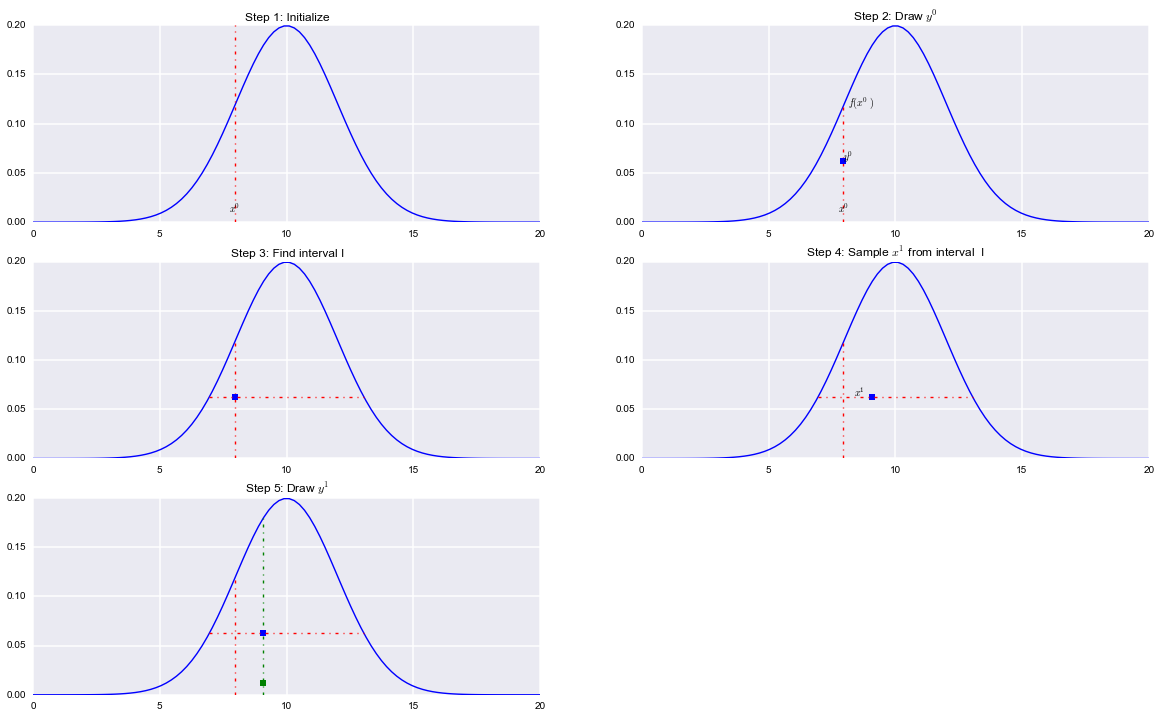This is workable assuming we can find the interval $I$. What about the multimodal case ?

In :
mu1=3; mu2=10; sigma1=1; sigma2=2; l1=.20; l2=.70;

normal = 1./np.sqrt(2*np.pi*sigma2**2)

fun=lambda x: l1*norm.pdf(x, mu1, sigma1)+l2*norm.pdf(x, mu2, sigma2)

In :
sns.set()
x = np.linspace(0,20, 100)

plt.figure(figsize=[20,12])

plt.subplot(2,2,1)
plt.plot(x, fun(x), 'b')

np.random.seed(16)
x0=np.random.uniform(low=0, high=20, size=1)
plt.plot( [x0 ,x0], [0, fun(x0)], 'r-.')
plt.title('Step 1: Initialize')
plt.annotate( '$x^{0}$', [x0+0.1,.001], xytext=None, xycoords='data',
textcoords='data', arrowprops=None)

plt.subplot(2,2,2)
plt.plot(x, fun(x), 'b')

plt.annotate( '$x^{0}$', [x0,.001], xytext=None, xycoords='data',
textcoords='data', arrowprops=None)
plt.annotate( '$f(x^0)$', [x0,fun(x0)], xytext=None, xycoords='data',
textcoords='data', arrowprops=None)

plt.plot( [x0 ,x0], [0, fun(x0)], 'r-.')
plt.title('Step 2: Draw $y^{0}$')
y0=np.random.uniform(low=0, high=fun(x0), size=1)
plt.plot( [x0,x0], [y0, y0], 'bs')

plt.annotate( '$y^{0}$', [10.5,.15], xytext=None, xycoords='data',
textcoords='data', arrowprops=None)

plt.subplot(2,2,3)
plt.plot(x, fun(x), 'b')
plt.plot( [x0 ,x0], [0, fun(x0)], 'r-.')
plt.plot( [x0,x0], [y0, y0], 'bs')
plt.plot( [5.7,14.2] , [y0, y0], 'r-.')
plt.plot( [1.3,5.1] , [y0, y0], 'r-.')
plt.title('Step 3: Find interval I')

plt.subplot(2,2,4)
plt.plot(x, fun(x), 'b')
plt.plot( [x0 ,x0], [0, fun(x0)], 'r-.')
plt.plot( [9,9], [y0, y0], 'bs')
plt.plot( [5.7,14.2] , [y0, y0], 'r-.')
plt.plot( [1.3,5.1] , [y0, y0], 'r-.')
plt.title('Step 4: Sample $x^{1}$ from interval  I')
plt.annotate( '$x^{1}$', [9.5,y0], xytext=None, xycoords='data',
textcoords='data', arrowprops=None)

Out:
<matplotlib.text.Annotation at 0x131562090>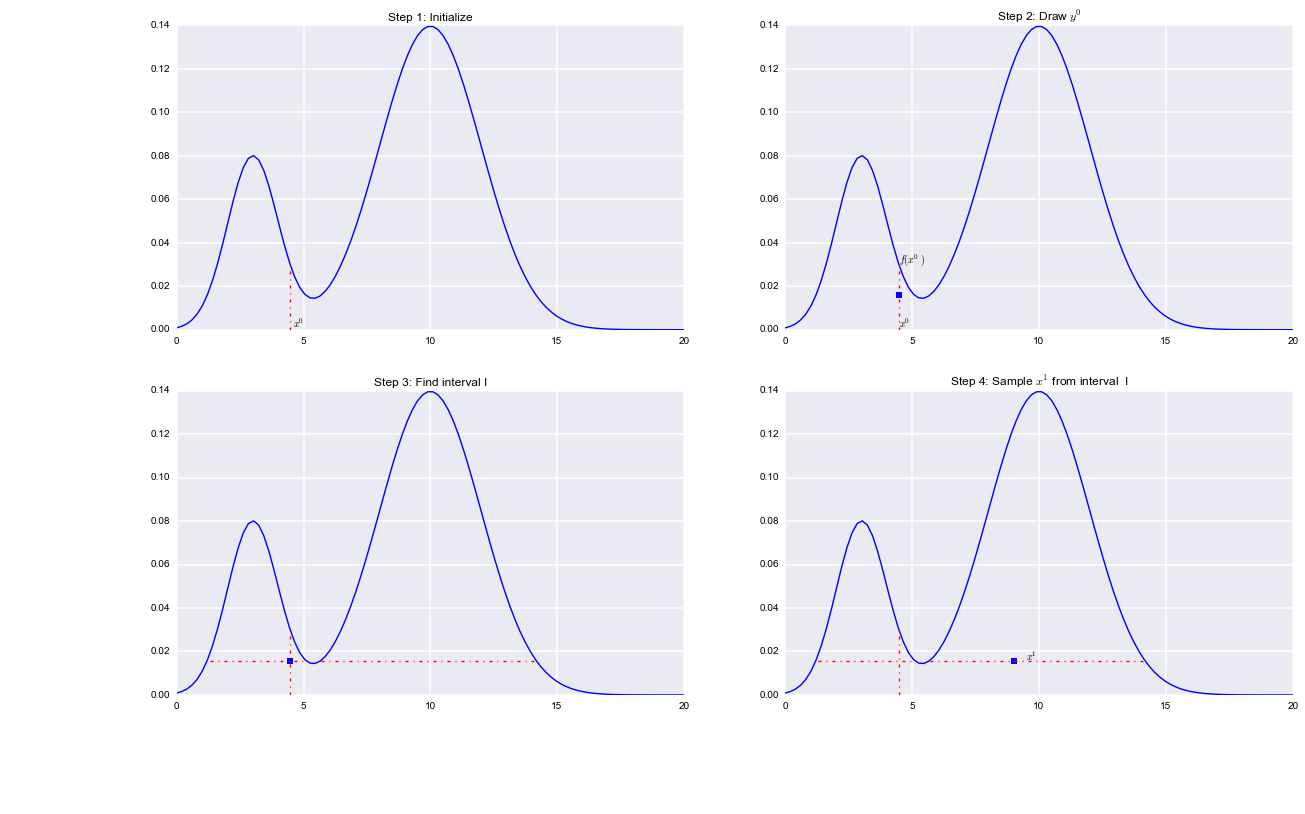The difficulty lies in steps 3 and 4. If you know the inverse of f(x) then you could set L = inf(S) (lower bound) and R = sup(S) (upper bound) as it is done in the unimodal example abpove. However in practice finding this region is not easy and there are various ways to do steps 3 and 4.

### Methods to Select the Univariate Window¶

There are a few different methods in the univariate case to select the Interval in Step 3 of the general procedure.

1. Find the ideal bounds as described above in unimodal case
2. If the range of $f(x)$ is bounded, then set the bounds of $f$ as your interval (L, R), draw $x$ from this interval and then reject/accept if it is below or above $f(x)$.
3. A procedure known as "Stepping Out" and
4. A procedure referred to as "Doubling."

(1) and (2) are usually infeasible for different reasons. (1) is infeasible because it's in general not easy to find the inf and sup of arbitrary regions of f(x). (2) is infeasible because in many cases the region S is much smaller than the bounded range of f(x) and sampling uniformly from the range of f(x) would be an inefficient way to sample from I. Let's concentrate on the other two methods.

#### Reject/accept sampling¶

In :
x = np.linspace(0,20, 100)

# number of samples
n=50000

vk=0

# randomly select x0
x_prev = np.random.uniform(low=0, high=17)

print x_prev
xsmp=[]

for k in np.arange(0,n):

if k<vk:
fig=plt.figure()
fig=plt.plot(x, fun(x))
fig=plt.plot( [x_prev ,x_prev], [0, fun(x_prev)], 'r-.')

y_next = np.random.uniform(low=0, high=fun(x_prev))

# ACCEPT REJECT
accept=False
while accept==False:
# NOW SAMPLE FROM ANYWHERE ON THE GREEN LINE
x_prop= np.random.uniform(low=0, high=20)

if k<vk:
fig=plt.plot( [x_prev,x_prev], [y_next, y_next], 'ks')
fig=plt.plot( [0,20], [y_next, y_next])
fig=plt.plot( [x_prop, x_prop], [y_next, y_next], 'bs')
#plt.title("Ising Model: {} Iterations".format(sample + 1))

display.clear_output()
display.display(plt.gcf())

if k<vk:
print raw_input('May I continue your majesty? ')

if y_next <=fun(x_prop):
if k<vk: fig=plt.plot( [x_prop, x_prop], [y_next, y_next], 'ro', ms=13)
x_prev = x_prop
xsmp.append(x_prop)
accept=True
else:
if k<vk: fig=plt.plot( [x_prop, x_prop], [y_next, y_next], 'ko', ms=12)
#print accept

if k<vk:
display.clear_output()
display.display(plt.gcf())
print raw_input('May I continue your majesty2? ')
plt.close()

#

9.51869303539

In :
plt.hist(xsmp,bins=50, alpha=0.3, normed=True);
#sns.kdeplot(xsmp)
plt.xlim( [0,20])
plt.plot(x, fun(x))

print len(xsmp)

50000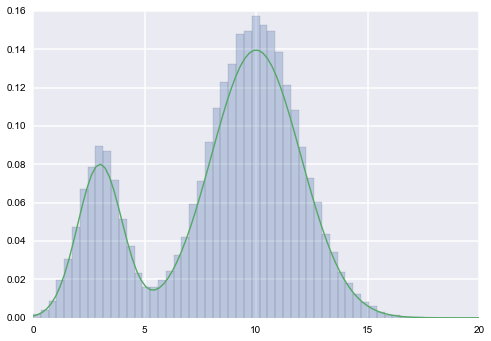#### Stepping Out¶

The idea behind stepping out is that you expand your interval by fixed widths $w$ until your endpoints are outside of S. The full algorithm is as follows:

• Set w = width of your interval expansions
• draw u, v ~ Unif(0,1)
• set L = $x^{(0)} - w u, R = L + w$ (so $x^{(0)}$ lies in [L, R] )
• while y < f(L) (here's where we extend left interval)
• L = L - w
• while y < f(R) ( here's where we extend the right interval)
• R = R + w

The final interval will be larger than $S$. We will later see how we accept/reject to ensure our samples are from within $S$.

In [ ]:
## STEPPING OUT EXAMPLE
w=1.0

x = np.linspace(0,20, 100)
vk=1
L=0; R=0;
# number of samples
n=5000
0
0

# randomly select x0
x_prev = np.random.uniform(low=0, high=17)

print x_prev
xsmp=[]

for k in np.arange(0,n):

if k<vk:
fig=plt.figure()
fig=plt.plot(x, fun(x))
fig=plt.plot( [x_prev ,x_prev], [0, fun(x_prev)], 'r-.')

y_next = np.random.uniform(low=0, high=fun(x_prev))

# NOW SAMPLE FROM ANYWHERE ON THE GREEN LINE
# STEPPING OUT
if k<vk:
fig=plt.plot( [x_prev,x_prev], [y_next, y_next], 'ks')

q=0
U = np.random.rand()
L=x_prev-U*w; R=x_prev+w*(1.0-U)
while fun(x_prev-q*w)>y_next:
q +=1
L = x_prev-q*w

if k<vk: fig=plt.plot( [L,R], [y_next, y_next], 'r.')
if k<vk: display.clear_output()
if k<vk: display.display(plt.gcf())

if k<vk:print raw_input('May I continue your majesty0a?')
q=0
R=x_prev+(1-U)*w
while fun(x_prev+q*w)>y_next:
q +=1
R = x_prev+q*w

if k<vk: fig=plt.plot( [L,R], [y_next, y_next], 'r.')
if k<vk: display.clear_output()
if k<vk: display.display(plt.gcf())

if k<vk: print raw_input('May I continue your majesty0b?')

x_prop= np.random.uniform(low=L, high=R)

if k<vk:

fig=plt.plot( [x_prop, x_prop], [y_next, y_next], 'bs')
#plt.title("Ising Model: {} Iterations".format(sample + 1))

display.clear_output()
display.display(plt.gcf())

if k<vk:
print raw_input('May I continue your majesty? ')

accept=False
while accept==False:
if y_next <fun(x_prop):
if k<vk: fig=plt.plot( [x_prop, x_prop], [y_next, y_next], 'ro', ms=13)
x_prev = x_prop
xsmp.append(x_prop)
accept = True
else:
if k<vk: fig=plt.plot( [x_prop, x_prop], [y_next, y_next], 'ko', ms=12)
x_prop= np.random.uniform(low=L, high=R)

if k<vk:
display.clear_output()
display.display(plt.gcf())
print raw_input('May I continue your majesty2? ')
plt.close()

#

---------------------------------------------------------------------------
KeyboardInterrupt                         Traceback (most recent call last)
<ipython-input-14-ab094632cdf4> in <module>()
45         if k<vk: display.display(plt.gcf())
46
---> 47         if k<vk:print raw_input('May I continue your majesty0a?')
48     q=0
49     R=x_prev+(1-U)*w

/Users/pprotopapas/anaconda/lib/python2.7/site-packages/IPython/kernel/zmq/ipkernel.pyc in <lambda>(prompt)
353         # raw_input in the user namespace.
354         if content.get('allow_stdin', False):
--> 355             raw_input = lambda prompt='': self._raw_input(prompt, ident, parent)
356             input = lambda prompt='': eval(raw_input(prompt))
357         else:

/Users/pprotopapas/anaconda/lib/python2.7/site-packages/IPython/kernel/zmq/ipkernel.pyc in _raw_input(self, prompt, ident, parent)
774             except KeyboardInterrupt:
775                 # re-raise KeyboardInterrupt, to truncate traceback
--> 776                 raise KeyboardInterrupt
777             else:
778                 break

KeyboardInterrupt: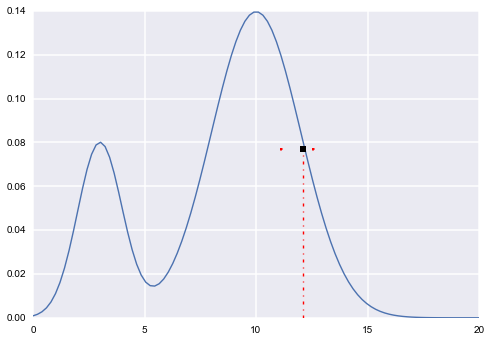In :
plt.hist(xsmp,bins=50, alpha=0.3, normed=True);
#sns.kdeplot(xsmp)
plt.xlim( [0,20])
plt.plot(x, fun(x))

print len(xsmp)
#plt.acorr(xsmp-np.mean(xsmp))

4141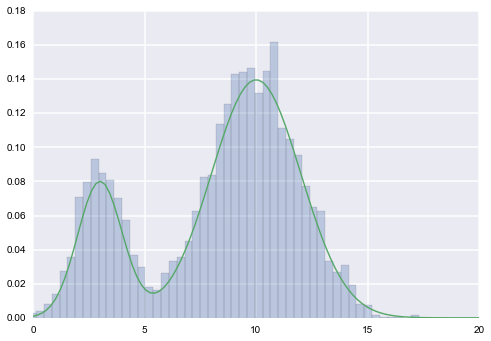#### Doubling¶

The idea behind doubling is that you expand your interval by doubling it's current length until endpoints are outside of S. The full algorithm is as follows:

• Set w = width of your initial interval
• draw u ~ Unif(0,1)
• set L = $x_0 - w \, u, R = L + w$ (so $x_0$ lies in [L, R] )

• until { y > f(R) and y > f(L) } ( here's where we extend the interval by doubling)

• flip a coin
• if heads then L = L - (R - L) otherwise R = R + (R - L)

Note that we double the length by adding a segment of the same size as our current size randomly to either the L or R endpoint. We do this regardless of whether that endpoint is already outside of S. We only stop when both sides are outside $S$.

### Methods to Sample from the Univariate Window¶

#### Acceptible Proposals¶

Once we've obtained our interval $I$, we want to sample from it. However as constructed $I$ is likely to be larger than the actual set of acceptable proposals. What are the set of acceptable proposals? They have to satisfy the following criteria:

1. They lie within $I$
2. They lie within $S$
3. They satisfy detail balance
• Let's call our current sample $x^{(0)}$
• Let's call a possible proposal x*
• x would be an acceptable proposal if $x_0$ would be an acceptable proposal for x if x* were the current sample

We can call our set of acceptable proposals for a given $x_0, A$.

#### Options¶

There are a few possible methods to sample from the interval.

1. We can repeatedly sample from within $I$ until we obtain a point within $S$
2. We can try a procedure known as "shrinkage"
3. If we used the doubling procedure we need an alternative "shrinkage" procedure

Repeatedly sampling from within $I$ as in 1), may not be efficient (especially if $S$ is much smaller than $I$). Let's then explore the "shrinkage" procedures.

#### Conventional Shrinkage Procedure¶

The idea behind the shrinkage procedure is that you sample from your interval, if the sample's not in $S$, make that point the new endpoint of your interval decreasing the length of your interval. Otherwise keep the sample and go back to the interval selection problem.

• start with interval $I = (L, R)$
• current sample is $x^{(k)}$ and $y^{(k)}$
• repeat until loop exits
• sample $x^{*}$ uniformly from (L, R)
• if $y^{(k)}< f(x^{*})$
• accept x* and end loop
• else
• if $x^{*} < x^{(k)} \rightarrow L = x^{*}$
• if $x^{*} > x^{(k)} \rightarrow R = x^{*}$

#### Shrinkage Procedure for Doubling¶

The conventional shrinkage procedure doesn't work for intervals $I$ generated by doubling because it's possible for a state to be within the intersection of $I$ and $S$ but not be an acceptable proposal. So we need to create a special shrinkage procedure that weeds out unacceptable proposals:

• start with interval $I = (L, R)$, current $X$ sample $x_0$ and $Y$ sample $y$
• proposal x*
• D = false, w = estimate of slice width
• repeat while (R-L) > 1.1*w
• M = (R + L)/2
• if ($x_0$ >= M and x < M) or ($x_0$ < M and x >= M) then D = true
• if x* < M then R = M otherwise L = M
• if y >= f(L) and y >= f(R) and D
• If the x* isn't rejected accept.

### Revisiting the Univariate Slice Sampling Algorithm¶

With all that background, our univariate slice sampling algorithm should be clear

• Pick an initial point $x_0$ from our posterior
• Draw $y_0$ from U(0, f($x_0$))
• Repeat for N samples
• Select the interval (e.g. doubling, stepping out, etc)
• Sample $x_i$ from that interval (e.g. shrinkage)
• Draw $y_i$ from U(0, f($x_i$))

## Revisiting Data Augmentation¶

Let's look a bit more closely at Data Augmentation and it's relationship to Slice Sampling. If you recall the exact procedure for data augmentation is as follows.

You're given $X$ from which you wish to sample. Sampling from them is difficult but with the addition of some auxilliary variables $Y$ you're able to sample from the joint probability p(X,Y) so that :

1. the conditionals X|Y and Y|X are easy to sample
2. the marginal p(X) from the joint p(X,Y) matches your target distribution.
3. You can then use Gibbs Sampling to generate samples for the joint distribution and keep the X samples

Now let's look more closely at our slice sampling procedure.

• We're given X
• We add an auxiliary variable Y
• The marginals are easy to sample
• Y|X ~ Unif(0,f(x))
• X|Y = 1 if f(x) > y and 0 otherwise
• the marginal of X matches our target distribution by construction
• we use Gibbs sampling to generate our samples

IT's A DATA AUGMENTATION PROCEDURE! The only difference is that we don't explicitly know our joint distribution.

## Multivariate Slice Sampling¶

On casual inspection it would seem that the slice sampling algorithm would generalize very easily to the multivariate case. If we look at our general univariate algorithm:

1. Inititalize by randomly selecting $x^{(0)}$
2. Draw $y^{(k)}$ from $U(0, f(x^{(k)}))$
3. Find an interval I = (L, R) around $x^{k}$ corresponding to $S = \{x\, s.t.\, f(x) > f(x^{k}) \}$
4. Draw $x^{k+1}$ from U(I)

A natural extension to $R^n$ would be:

1. Inititalize by randomly selecting $\hat{x}^{(0)}$
2. Draw $y^{(k)}$ from $U(0, f(x^{(k)}))$
3. Find a hyperrectangle K = $\{(L_1,R_1)\times \ldots \times(L_n, R_n)\}$ around $\hat{x}^{k}$ corresponding to $S = \{\hat{x}\, s.t.\, f(\hat{x}) > f(\hat{x}^{k}) \}$
4. Draw $\hat{x}^{k+1}$ uniformly from K

Step 1 is not difficult and generalizes easily. Step 2 is difficult. We ideally want the minimum hyperrectangle containing $S$, but in practice this is just as (if not more) difficult as finding the ideal interval $I$ in the univariate case. Moreover some of the techniques that we described in the univariate case have difficulties in the multivariate case.

• Stepping out is difficult because an $n$ dimensional hyperrectangle has $2^n$ vertices. Checking individual vertices can be a computationally expensive problem. In addition, stepping out individually in $n$ directions can be computationally expensive as well.

• Accept/reject on a global region (in the case where your distribution is bounded) is even less efficient in multiple dimensions than it is in one.

We outline a simple solution that gives up some of the natural advantages of univariate slice sampling but does solve some of the basic issues for multivariate slice sampling. It depends on having some prior knowledge of the appropriate hyperectagle width in each dimension.

#### Hyperrectangle multivariate slices sample algorithm¶

• start with $x^{k}$ and $\hat{w}$ -- the prior widths
• $y^{k} \sim Unif(0,f(x^{k}))$
• for $i = 1 \ldots n$ (setting hyperrectangle around $x^{k}$)
• $u_i \sim Unif(0,1)$
• $L_i = x^{k}_i - w_i u_i$
• $R_i = L_i + w_i$
• loop until:
• for $i = 1 \ldots n$
• $u_i \sim Unif(0,1)$
• $x^{*}_i = Unif(L_i, R_i)$
• if $y^{(k)}< f(x^{*})$
• accept x* and end loop
• else
• for $i = 1 \ldots n$
• if $x^{*}_i < x^{(k)}_i \rightarrow L_i = x^{*}_i$
• if $x^{*}_i > x^{(k)}_i \rightarrow R_i = x^{*}_i$

It should be noted that because we don't use a method like doubling or setting out, we gain an increased sensitivity to step-size (via our dependence on the width vector $\hat{w}$ and lose part of the advantage slice sampling held over Metropolis-Hastings. Width is now a tunable parameter.

In :
def mvslice(pdf, x0, widths, sampleSize=1000, dims=2, burnin=0, thin=0):

"""

:param pdf:  function we're trying to sample
:param x0:   inital point
:param widths:  prior for widths of our hyperrectangle
:param sampleSize:  number of samples to generate
:param dims:  dimension of our multivariate space
:param burnin:  number of samples to get rid of at beginning
:param thin:   number of samples to keep
"""
y0 = np.random.uniform(low=0, high=pdf(x0))
samples = []

# get hyperrectangle
rectUnifs = np.random.uniform(size=dims)
rectLefts = x0 - widths*rectUnifs
rectRights = rectLefts + widths

# Get our samples
for i in range(sampleSize):

while (True):

# new proposal
xstarUnifs = np.random.uniform(size=dims)
xstar = rectLefts + xstarUnifs*(rectRights - rectLefts)

if y0 < pdf(xstar):
break
else:

# update rectangle
for j in range(dims):

if xstar[j] < x0[j]:
rectLefts[j] = xstar[j]
else:
rectRights[j] = xstar[j]

# save our sample
samples.append(xstar)

# Our last sample x0 is now our proposal
x0 = xstar

# Get our new y0 for next step
y0 = np.random.uniform(low=0, high=pdf(x0))

# reset our rectangle
rectLefts = x0 - widths*rectUnifs
rectRights = rectLefts + widths

return samples

pdf = lambda x: np.e**(-(x**2)/2 - ((-x)**2)/2)
X = mvslice(pdf,[0,0], [10,10], sampleSize=10000)
Xs = [a for a in X]
Ys = [a for a in X]

In :
plt.scatter(Xs, Ys, alpha=0.1, s=2.0)
sns.kdeplot(np.array(Xs), np.array(Ys))

Out:
<matplotlib.axes.AxesSubplot at 0x10fdd8090>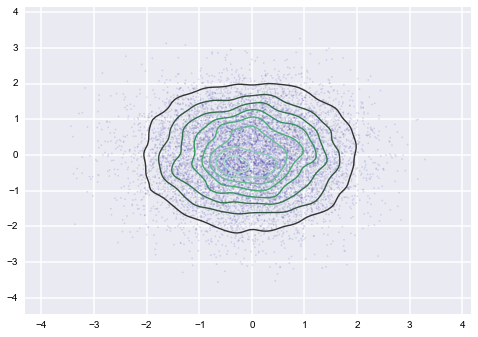In [ ]: